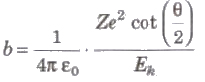Dalton’s Atomic Theory

All elements are consists of very small invisible particles, called atoms. Atoms of same element are exactly same and atoms of different element are different.

Thomson’s Atomic Model

Every atom is uniformly positive charged sphere of radius of the order of 10-10 m, in which entire mass is uniformly distributed and negative charged electrons are embedded randomly. The atom as a whole is neutral.Limitations of Thomson’s Atomic Model

1. It could not explain the origin of spectral series of hydrogen and other atoms.

2. It could not explain large angle scattering of α – particles.

Rutherford’s Atomic Model

On the basis of this experiment, Rutherford made following observations

(i) The entire positive charge and almost entire mass of the atom is concentrated at its centre in a very tiny region of the order of 10-15 m, called nucleus.

(ii) The negatively charged electrons revolve around the nucleus in different orbits.

(iii) The total positive charge 011 nucleus is equal to the total negative charge on electron. Therefore atom as a overall is neutral.

(iv) The centripetal force required by electron for revolution is provided by the electrostatic force of attraction between the electrons and the nucleus.Distance of Closest Approach

ro = 1 / 4π εo . 2Ze2 / Ek

where, Ek = kinetic energy of the cc-particle.

Impact Parameter

The perpendicular distance of the velocity vector of a-particle from the central line of the nucleus, when the particle is far away from the nucleus is called impact parameter.

Impact parameterwhere, Z = atomic number of the nucleus, Ek = kinetic energy of the c-particle and θ = angle of scattering.

Rutherford’s Scattering Formulawhere, N(θ) =number of c-particles, Ni = total number of α-particles reach the screen. n = number of atoms per unit volume in the foil, Z = atoms number, E = kinetic energy of the alpha particles and t = foil thicknessLimitations of Rutherford Atomic Model

(i) About the Stability of Atom According to Maxwell’s electromagnetic wave theory electron should emit energy in the form of electromagnetic wave during its orbital motion. Therefore. radius of orbit of electron will decrease gradually and ultimately it will fall in the nucleus.

(ii) About the Line Spectrum Rutherford atomic model cannot explain atomic line spectrum.

Bohr’s Atomic Model

Electron can revolve in certain non-radiating orbits called stationary or bits for which the angular momentum of electron is an integer multiple of (h / 2π)

mvr = nh / 2π

where n = I, 2. 3,… called principle quantum number.

The radiation of energy occurs only when any electron jumps from one permitted orbit to another permitted orbit.

Energy of emitted photon

hv = E2 – E1

where E1 and E2are energies of electron in orbits.

Radius of orbit of electron is given by

r = n2h2 / 4π2 mK Ze2 ⇒ r ∝ n2 / Z

where, n = principle quantum number, h = Planck’s constant, m = mass of an electron, K = 1 / 4 π ε, Z = atomic number and e = electronic charge.

Velocity of electron in any orbit is given by

v = 2πKZe2 / nh ⇒ v ∝ Z / n

Frequency of electron in any orbit is given by

v = KZe2 / nhr = 4π2Z2e4mK2 / n3 h3

⇒ v prop; Z3 / n3

Kinetic energy of electron in any orbit is given by

Ek = 2π2me4Z2K2 / n2 h2 = 13.6 Z2 / n2 eV

Potential energy of electron in any orbit is given by

Ep = – 4π2me4Z2K2 / n2 h2 = 27.2 Z2 / n2 eV

⇒ Ep = ∝ Z2 / n2

Total energy of electron in any orbit is given by

E = – 2π2me4Z2K2 / n2 h2 = – 13.6 Z2 / n2 eV

⇒ Ep = ∝ Z2 / n2

Wavelength of radiation emitted in the radiation from orbit n2 to n1 is given byIn quantum mechanics, the energies of a system are discrete or quantized. The energy of a particle of mass m is confined to a box of length L can have discrete values of energy given by the relation

En = n2 h2 / 8mL2 ; n < 1, 2, 3,…

Hydrogen Spectrum Series

Each element emits a spectrum of radiation, which is characteristic of the element itself. The spectrum consists of a set of isolated parallel lines and is called the line spectrum.Hydrogen spectrum contains five series

(i) Lyman Series When electron jumps from n = 2, 3,4, …orbit to n = 1 orbit, then a line of Lyman series is obtained.

This series lies in ultra violet region.

(ii) Balmer Series When electron jumps from n = 3, 4, 5,… orbit to n = 2 orbit, then a line of Balmer series is obtained.

This series lies in visual region.

(iii) Paschen Series When electron jumps from n = 4, 5, 6,… orbit to n = 3 orbit, then a line of Paschen series is obtained.

This series lies in infrared region

(iv) Brackett Series When electron jumps from n = 5,6, 7…. orbit to n = 4 orbit, then a line of Brackett series is obtained.

This series lies in infrared region.

(v) Pfund Series When electron jumps from n = 6,7,8, … orbit to n = 5 orbit, then a line of Pfund series is obtained.

This series lies in infrared region.

Wave Model

It is based on wave mechanics. Quantum numbers are the numbers required to completely specify the state of the electrons.

In the presence of strong magnetic field, the four quantum number are

(i) Principle quantum number (n) can have value 1,2, … ∞

(ii) Orbital angular momentum quantum number l can have value 0,1, 2, … ,(n – 1).

(iii) Magnetic quantum number (me) which can have values – I to I.

(iv) Magnetic spin angular momentum quantum number (ms) which can have only two value + 1 / 2.